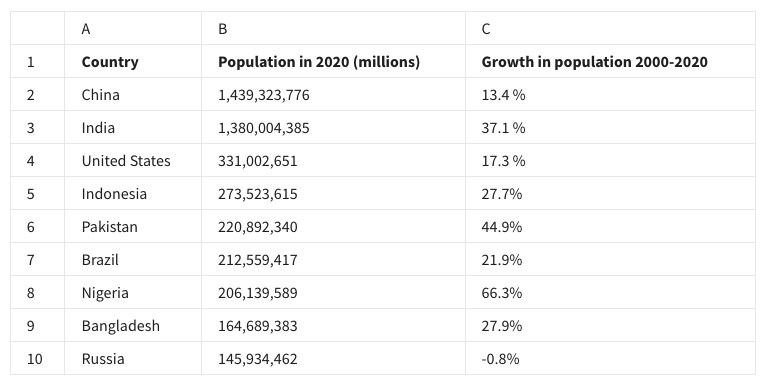# analyze data to answer questions weekly challenge 3

• expanded
• localized
• modified
• summarized

• VALUE
• FIGURE
• CONVERT
• DIGIT

• True
• False

#### 4. Fill in the blank: When writing a function, a data analyst wraps a table array in dollar signs. This is an _____ , which is used to lock the array so rows and columns don’t change if the function is copied.

• accurate reference
• absolute reference
• arbitrary reference
• authentic reference

#### 5. The following is a selection from a spreadsheet:#### To search for the growth in population in Indonesia, what is the correct VLOOKUP syntax?

• =VLOOKUP(“Indonesia”, A2:C10, 3, false)
• =VLOOKUP(Indonesia, A2*C10, 3, false)
• =VLOOKUP(“Indonesia”, A2:C10, 2, false)
• =VLOOKUP(Indonesia, A2:C10, 2, false)

• True
• False

#### 7. A data analyst writes a query that asks a database to return the number of rows in a specified range. Which function do they use?

• COUNT DISTINCT
• RANGE
• COUNT
• RETURN RANGE

#### 8. Fill in the blank: In a SQL statement, the _____ is the name of the segment that executes first. Select all that apply.

• central select
• inner query
• central query
• inner select

## Shuffle Q/A 1

#### 9. In data analytics, what is the process of gathering data from multiple sources and combining it into a single, summarized collection?

• Data composition
• Data aggregation
• Data grouping
• Data mapping

#### 10. A data analyst is performing numerical calculations on the data in their spreadsheet. Ahead of these calculations, they use the VALUE function. Why might they do this?

• To get a list of all the distinct numbers in the data
• To convert the numbers in the data from text to numerical values
• To sum up all the numbers in the spreadsheet
• To find the average of all the numbers in the spreadsheet